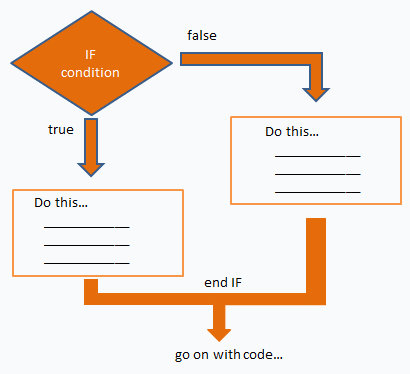# if statement - Branches

The if statement evaluates a logical expression and executes a group of statements when the expression is true.

The optional elseif and else keywords provide for the execution of alternate groups of statements.

An end keyword, which matches the if, terminates the last group of statements.

This type of structure is also known as branches or 'branching'.

The groups of statements are delineated by the four keywords (no braces or
brackets are involved).

The general form of the statement is:

if expression1
statements1
...
elseif expression2
statements2
...
else
statements3
...
endIt is important to understand how relational operators and if statements work with matrices.

When you want to check for equality between two variables, you might use if A == B ...

This '==' code is fine, and it does what you expect when A and B are scalars.

But when A and B are matrices, A == B does not test if they are equal, it tests where they are equal; the result is another matrix of 0’s and 1’s showing element-by-element equality.

In fact, if A and B are not the same size, then A == B is an error.

The proper way to check for equality between two variables is to use the
isequal function:

if isequal(A,B) ...

Here's an example code:

if m == n
a(m,n) = 3;
elseif abs(m-n) == 3
a(m,n) = 1;
else
a(m,n) = 0;
end

If m equals n, then a(m,n) becomes 3, and the routine continues after the end.

If not, the routine tests if abs(m-n) equals 3. If it does, then a(m,n) becomes 1, and the routine continues after the end.

In any other case a(m,n) becomes 0, and the routine continues after the end.

Several functions are helpful for reducing the results of matrix comparisons to scalar conditions for use with if, including:

isequal
isempty
all
any

From 'if statement' to home

From 'if statement' to 'Matlab Code'